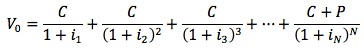### Seeing is believing!

Before you order, simply sign up for a free user account and in seconds you'll be experiencing the best in CFA exam preparation.

##### Subject 2. Relationships between the Values of a Callable or Putable Bond, Straight Bond, and Embedded Option
A callable bond has two disadvantages for an investor:

• If it is effectively called, the investor will have to invest in another bond, yielding a lower rate.
• A callable bond has the unpleasant property for an investor to appreciate less than a normal similar bond when interest rates fall.
• Therefore, an investor will be willing to buy such a bond at a lower price than a comparable option-free bond.

If we value two bonds:

• An option-free 5% 4-year bond is valued at 102.555.
• A 5% 4-year bond callable at any time since the end of year 1 is valued at 101.516.

The difference between these two values is attributed to the embedded call option. Therefore, the call option is worth 102.555 - 101.516 = 1.039% of principal. This value is positive for the issuer and negative for investors, since it is the issuer who benefits from the option.

Value of Callable Bond = Value of Option-Free Bond - Value of Call Option

By the same token, a putable bond trades at a higher price than a comparable option-free bond. For example, consider the formula for the value of a putable bond:

Value of Putable Bond = Value of Option-free Bond + Value of Put Option.

The general expression, incorporating the previous two formulas would be:

Value of Bond with Embedded Options = Value of Option-Free Bond + Value of Options Granted to Investors - Value of Options Granted to the Issuer

Valuation of Default-Free and Option-Free Bonds

If we know any one of spot rates, par rates and forward rates we can determine the other two.

Assume the following par rates:
Year | Par Rate (%)
1 | 2%
2 | 2.2%
3 | 2.5%

The 1-year spot rate is 2%.
For the 2-year spot rate S2: 2.2/1.02 + 102.2/(1+S2)2 = 100, S2 = 2.2022%

Assume the following spot rates:
Year | Spot Rate (%)
1 | 2%
2 | 2.2%
3 | 2.5%

The 1-year forward rate 1 year from now F1,1 = (1+0.022)2/(1+0.02) - 1 = 2.4%
The 1-year forward rate 2 year from now F2,1 = (1+0.025)2/(1+0.022)2 = 3.5%

The arbitrage-free approach has three steps:

• Take each individual cash flow of a coupon as a stand-alone zero-coupon bond. Each cash flow is the face value of the corresponding zero.
• Value each zero-coupon bond by discounting its cash flow at the corresponding spot rate.
• Add up the value of each zero to calculate the total value of the zero-coupon bond portfolio.User Comment
pingpong The call feature is positive for the issuer of the bond as it allows the issuer to essentially refinance debt at more favorable terms when interest rates fall. For the investor, on the other hand, this represents a drawback as it causes the price behavior of this security to exhibit negative convexity when interest rate levels fall. This limits the capital appreciation potential of the bonds when interest rates fall. Investors are usually compensated for this drawback through a greater return potential as callable bonds are usually priced at a discount to other comparable non-callable fixed income securities.
TheCFAGuy the equation for the 1yr fwd 2yrs from now is wrong. should read:

F(2)(1) = (1+0.025)^3/(1+0.022)^2 = 3.1%

if you invest in the 2yr bond, EMH says at the end of those 2yrs you should be able to purchase a 1 yr bond and earn the equal return of a 3 year bond purchased today.

EMH applies because if investors suspect that the implied forward rate is too high they will buy more 3 year bonds, driving down the yield to a point where the implied rate looks more reasonable... or maybe they'll overreact and the implied forward rate will go 'too low' at which point more investors start buying 2yr bonds to roll into 1yr bonds
ashish100 Agreed with above